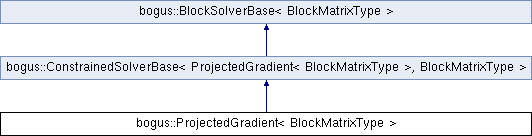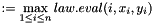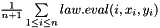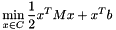So-Bogus A c++ sparse block matrix library aimed at Second Order cone problems
bogus::ProjectedGradient< BlockMatrixType > Class Template Reference

Projected Gradient iterative solver. More...

#include <ProjectedGradient.hpp>

Inheritance diagram for bogus::ProjectedGradient< BlockMatrixType >:## Public Types

typedef ConstrainedSolverBase
< ProjectedGradient,
BlockMatrixType >
Base

typedef Base::Scalar Scalar

typedef Base::GlobalProblemTraits GlobalProblemTraits

typedef LocalProblemTraits
< Base::BlockTraits::RowsPerBlock,
Scalar >
BlockProblemTraits

typedef BlockMatrixTraits
< BlockMatrixType >
BlockTraits

typedef Signal< unsigned, Scalar > CallBackType

## Public Member Functions

ProjectedGradient ()
Default constructor – you will have to call setMatrix() before using the solve() function.

ProjectedGradient (const BlockObjectBase< BlockMatrixType > &matrix)
Constructor with the system matrix.

template<typename NSLaw , typename RhsT , typename ResT >
Scalar solve (const NSLaw &law, const RhsT &b, ResT &x) const
Finds an approximate minimum for a constrained quadratic problem.

template<projected_gradient::Variant variant, typename NSLaw , typename RhsT , typename ResT >
Scalar solve (const NSLaw &law, const RhsT &b, ResT &x) const
Finds an approximate minimum for a constrained quadratic problem. More...

ProjectedGradientsetMatrix (const BlockObjectBase< BlockMatrixType > &matrix)
Sets the matrix M defining the quadratic objective function. More...

void setLineSearchIterations (const unsigned lsIterations)
Sets the maximum number of line-search iterations.

void setLineSearchOptimisticFactor (const Scalar lsOptimisticFactor)

void setLineSearchPessimisticFactor (const Scalar lsPessimisticFactor)

void setLineSearchArmijoCoefficient (const Scalar lsArmijoCoefficient)

void setDefaultVariant (projected_gradient::Variant variant)
Sets the variant that will be used when calling solve() without template arguments.

unsigned lineSearchIterations () const

Scalar lineSearchOptimisticFactor () const

Scalar lineSearchPessimisticFactor () const

Scalar lineSearchArmijoCoefficient () const

void useInfinityNorm (bool useInfNorm)
Sets whether the solver will use the infinity norm instead of the l1 one to compute the global residual from the local ones.

bool usesInfinityNorm () const

Scalar eval (const NSLaw &law, const ResT &y, const RhsT &x) const
Eval the current global residual as a function of the local ones. More...

void projectOnConstraints (const NSLaw &projector, VectorT &x) const
Projects the variable x on the constraints defined by projector.

void dualityCOV (const NSLaw &law, const RhsT &b, ResT &x) const
Compute associated change of variable (see NSLaw)

void setMaxIters (unsigned maxIters)
For iterative solvers: sets the maximum number of iterations.

unsigned maxIters () const

void setTol (Scalar tol)
For iterative solvers: sets the solver tolerance.

Scalar tol () const

CallBackTypecallback ()
Callback hook; will be triggered every N iterations, depending on the solver. More...

const CallBackTypecallback () const

const BlockObjectBase
< BlockMatrixType > &
matrix () const

## Protected Types

typedef Base::Index Index

## Protected Member Functions

void init ()
Sets up the default values for all parameters.

void updateScalings ()

## Protected Attributes

unsigned m_lsIters

Scalar m_lsOptimisticFactor

Scalar m_lsPessimisticFactor

Scalar m_lsArmijoCoefficient

projected_gradient::Variant m_defaultVariant

GlobalProblemTraits::DynVector m_scaling

bool m_useInfinityNorm
See useInfinityNorm(). Defaults to false.

const BlockObjectBase
< BlockMatrixType > *
m_matrix
Pointer to the matrix of the system.

unsigned m_maxIters
See setMaxIters()

Scalar m_tol
See setTol()

CallBackType m_callback

## Detailed Description

### template<typename BlockMatrixType> class bogus::ProjectedGradient< BlockMatrixType >

Projected Gradient iterative solver.

## Member Function Documentation

template<typename BlockMatrixType >
 CallBackType& bogus::BlockSolverBase< BlockMatrixType >::callback ( )
inherited

Callback hook; will be triggered every N iterations, depending on the solver.

Useful to monitor the convergence of the solver. Can be connected to a function that takes an unsigned and a Scalar as parameters. The first argument will be the current iteration number, and the second the current residual.

See Also
Signal< unsigned, Scalar >
 Scalar bogus::ConstrainedSolverBase< ProjectedGradient< BlockMatrixType > , BlockMatrixType >::eval ( const NSLaw & law, const ResT & y, const RhsT & x ) const
inherited

Eval the current global residual as a function of the local ones.

y should be such that y = m_matrix * x + rhs

Returns
the current residual err defined as follow :
• if m_useInfinityNorm is true, then err• else err :=template<typename BlockMatrixType>
 void bogus::ProjectedGradient< BlockMatrixType >::setLineSearchArmijoCoefficient ( const Scalar lsArmijoCoefficient )

Sets the objective decrease coefficient for linesearchs that use an Armijo exit criterion Should be in ]0,1[

template<typename BlockMatrixType>
 void bogus::ProjectedGradient< BlockMatrixType >::setLineSearchOptimisticFactor ( const Scalar lsOptimisticFactor )

Sets the amount by which the step size will be multiplied at the beginninf of each PG iteration. Should be greater than 1

template<typename BlockMatrixType>
 void bogus::ProjectedGradient< BlockMatrixType >::setLineSearchPessimisticFactor ( const Scalar lsPessimisticFactor )

Sets the amount by which the step size will be multiplied at the end of each line-search iterations. Should be in ]0,1[

template<typename BlockMatrixType>
 ProjectedGradient& bogus::ProjectedGradient< BlockMatrixType >::setMatrix ( const BlockObjectBase< BlockMatrixType > & matrix )

Sets the matrix M defining the quadratic objective function.

M should be symmetric, positive

template<typename BlockMatrixType>
template<projected_gradient::Variant variant, typename NSLaw , typename RhsT , typename ResT >
 Scalar bogus::ProjectedGradient< BlockMatrixType >::solve ( const NSLaw & law, const RhsT & b, ResT & x ) const

Finds an approximate minimum for a constrained quadratic problem.

Find an approximate solution towhere C is defined through the orthogonal projection operation on C by NSLaw::projectOnConstraint().

Returns
an evalutation of the optimality error computed using NSLaw::eval()
Template Parameters
 variant Which variant of the algorithm to use.

The documentation for this class was generated from the following file: